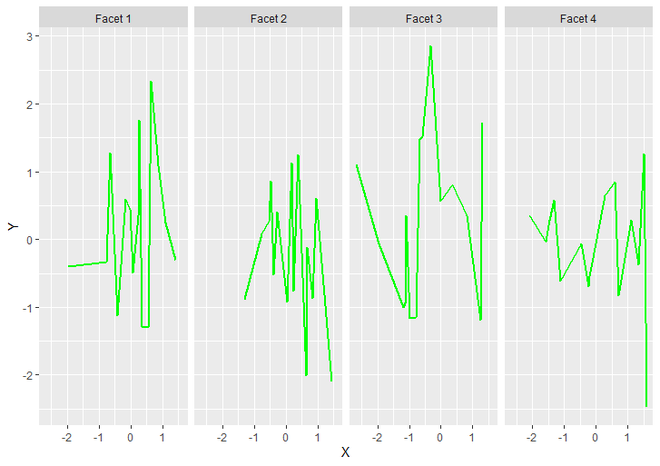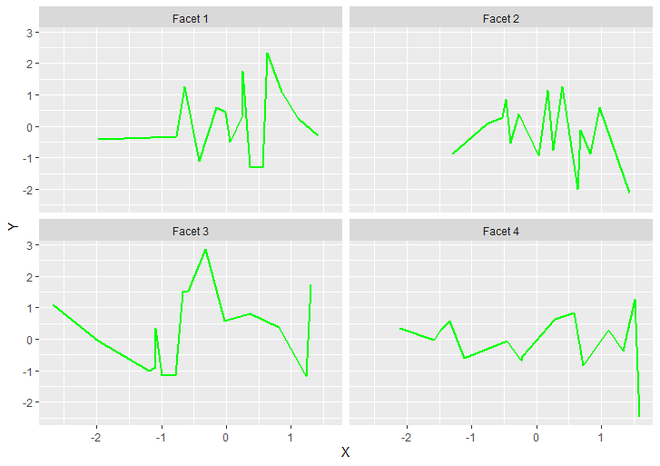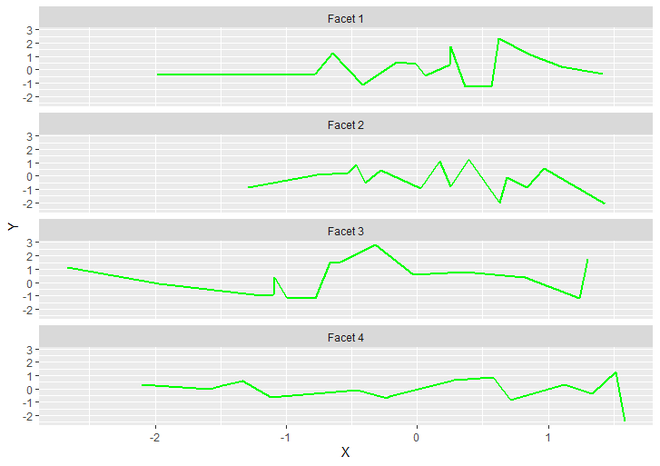# How to create a faceted line-graph using ggplot2 in R ?

• Last Updated : 24 Jun, 2021

In this article, we will see how to create a faceted line-graph using ggplot2 in R Programming Language.

In ggplot2, geom_line() function is used to create a line graph. Facets can be created after adding separate methods along with required parameters.

### Method 1: Using facet_grid()

First a dataframe is created, and then we create a faceted Line Graph by adding facet_grid() function to geom_line.

Syntax : facet_grid(facets, …)

Parameters :

• facets : this parameter is necessary to use with facet_grid. which specify the formula with the rows on LHS and columns on RHS. the dot parameter used in the formula to indicate that there should be no faceting on this dimension (row or column).
• … : facet_grid function has also many parameters such as scale, space, margins, shrink, etc . but they are not necessary to use. they all have some default values but if we want to change them than we can use them. But for faceting first parameter is enough.

Return : Facets on a Plot.

Syntax In Use :

facet_grid(row ~ column)

As in above syntax, we use Facets vector to indicate column and nothing for row. For specifying nothing, we use dot parameter like facet_grid(. ~ Facets). This will return vertical facets on the plot and to draw horizontal facets, we simply have to interchange the dot parameter and facets vector in facet_grid() function.

Example :

## R

 `# Load Library``library``(``"ggplot2"``) `` ` `# Create DataFrame``DF <- ``data.frame``(X = ``rnorm``(60),                                   ``                 ``Y = ``rnorm``(60),``                 ``Facets = ``c``(``"Facet 1"``, ``"Facet 2"``,``                            ``"Facet 3"``, ``"Facet 4"``))`` ` `# Create Faceted LineGraph with Verticle Facets.``ggplot``(DF, ``aes``(X, Y)) +                                     ``  ``geom_line``(color = ``"green"``, size = 1) +``  ``facet_grid``(. ~ Facets)`

Output:Faceted LineGraph with verticle facets using facet_grid

### Method 2: Using facet_wrap()

We can also create Faceted Line Graph using facet_wrap() function, which is generally better uses screen space than facet_grid() as it wraps a one dimensional sequence of panels into two dimensional. That means it creates 4 facets for our DataFrame as 2×2 manner. We also change the number of rows and columns of facets using its parameters.

Syntax : facet_wrap(facets, nrow, ncol, …)

Parameters :

• facets : same as facets parameter of facet_grid functionas we discussed above. it specify the formula with row at LHS and column at RHS i.e row ~ column.
• nrow : Number of rows of facets.
• ncol : Number of columns of facets.
• … : facet_wrap has also some parameters that doesn’t matter as much as they have it’s Default values. We can use them in some special case.

Return : Convert 1D sequence of facets into 2D.

Example :

## R

 `# Load Library``library``(``"ggplot2"``) `` ` `# Create a DataFrame for Plotting``DF <- ``data.frame``(X = ``rnorm``(60),                                   ``                 ``Y = ``rnorm``(60),``                 ``Facets = ``c``(``"Facet 1"``, ``"Facet 2"``,``                            ``"Facet 3"``, ``"Facet 4"``))`` ` `# Create a Faceted LineGraph using``# facet_wrap Function.``ggplot``(DF, ``aes``(X, Y)) +                                     ``  ``geom_line``(color = ``"green"``, size = 1) +``  ``facet_wrap``(. ~ Facets)`

Output:2×2 Faceted LineGraph using facet_wrap

By default, the output came as a grid for this one. But it can be changed using nrow and ncol parameter with an appropriate value.

Example :

## R

 `# Load Library``library``(``"ggplot2"``) `` ` `# Create a DataFrame for Plotting``DF <- ``data.frame``(X = ``rnorm``(60),                                   ``                 ``Y = ``rnorm``(60),``                 ``Facets = ``c``(``"Facet 1"``, ``"Facet 2"``, ``                            ``"Facet 3"``, ``"Facet 4"``))`` ` `# Create a Faceted LineGraph using facet_wrap ``# Function with changed number of rows.``ggplot``(DF, ``aes``(X, Y)) +                                     ``  ``geom_line``(color = ``"green"``, size = 1) +``  ``facet_wrap``(. ~ Facets, nrow = 4)`

Output:Faceted LineGraph using facet_wrap with different number of rows

My Personal Notes arrow_drop_up